# Inductance

## (6) The R-C circuit

• Consider a circuit containing a capacitor of capacitance C and a resistor R connected to a constant source of emf (battery) through a key (K) as shown below in the figure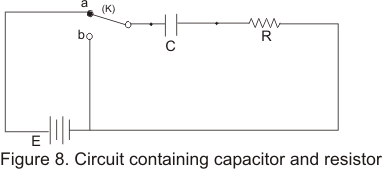• Source of EMF E can be included or excluded from circuit using this two way key

### (A) Growth of charge

• when the battery is included in the circuit by throwing the switch to a, the capacitor gradually begins to charge and because of this capacitor current in the circuit will vary with time
• There are two factors which contributes to voltage drop V across the circuit i.e. if current I flows through resistor R,voltage drop across the resistor is IR and if there is a charge Q on the capacitor then voltage drop across it would be Q/C
• At any instant, instantaneous potential difference across capacitor and resistor are
VR=IR and VC=q/C
Therefore total potential difference drop across circuit is
V=VR+VC=IR+q/C
Where V is a constant
• Now current is circuit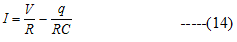Initially at time t=0 ,when the connection was made, charge on the capacitor q=0 and initial current in the circuit would be Imax=V/R ,which would be the steady current in the circuit in the absence of the capacitor
• As the charge q on the capacitor increase ,the term q/RC becomes larger and current decreases until it becomes zero .Hence for I=0

V/R=q/RC
or q=CV=Qf

where Qf is the final charge on the capacitor
• Again consider equation (14)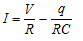we know that I=dq/dt
So,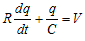rearranging this equation we get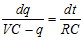Integrating this we get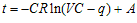Where A is the constant of integration
Now at t=0,q=0 So
A=CRlnCV
From this we have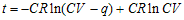As CV=Qf so,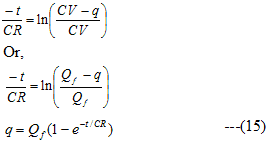Where Qf=CV as defined earlier is the final charge on the capacitor when potential difference across it becomes equal to applied to EMF
• Equation (15) represents the growth of charge on the capacitor and shows that it grows exponentially as shown below in the figure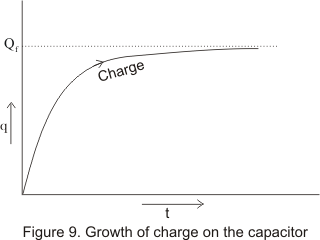• Now since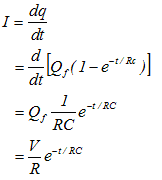Again Imax=V/R,so we have,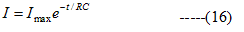Thus from equation (16) we see that current decreases exponentially from its maximum value Imax=V/R to zero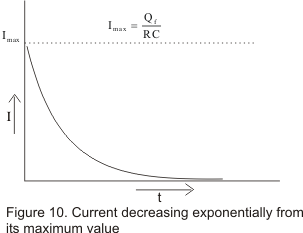• Quantity RC in equation (15) and (16) is called capacitive time constant of the circuit
τC=CR
• Smaller is the value of τC,charge will grow on the capacitor more rapidly.
• Putting t= τC=CR in equation (15)
q=Qf(1-e-1)
=6.32Qf
Thus τC of CR circuit is the time which the charge on capacitor grows from 0 to .632 of its maximum value

### (B) Decay of charge

• Again consider figure (8),by throwing the switch(S) to b,we can now exclude the battery from the circuit
• After the removal of external emf the charged capacitor now begins to discharge through the resistance R
• Putting V=0 in equation (14) we have
I=-q/RC
or dq/dt=-q/RC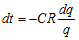On integrating this equation we get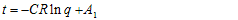Where A1 is the constant of integration .Initially at t=0,q=Qf ,since capacitor is fully charged thus
A1=CRlnQf
Hence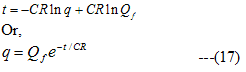This is the equation governing discharge of capacitor C through resistance R
• From equation (17) we see that charge on a capacitor decays exponentianally with time as shown below in the figure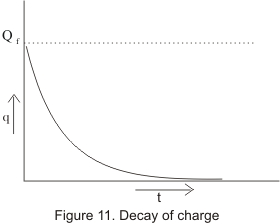• Current during discharge is obtained by differentiating the equation (17) so,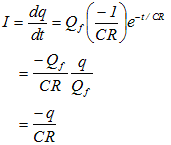Thus smaller the capacitive time constant ,the quicker is the discharge of the capacitor
• Putting t= τC=CR in equation (17)
We get
q=Qfe-1=Qf(.368)
Thus the capacitive time constant can also be defined as the time in which the charge on the capacitor decays from maximum to .368 of the maximum value

Note to our visitors :-

Thanks for visiting our website.
DISCLOSURE: THIS PAGE MAY CONTAIN AFFILIATE LINKS, MEANING I GET A COMMISSION IF YOU DECIDE TO MAKE A PURCHASE THROUGH MY LINKS, AT NO COST TO YOU. PLEASE READ MY DISCLOSURE FOR MORE INFO.